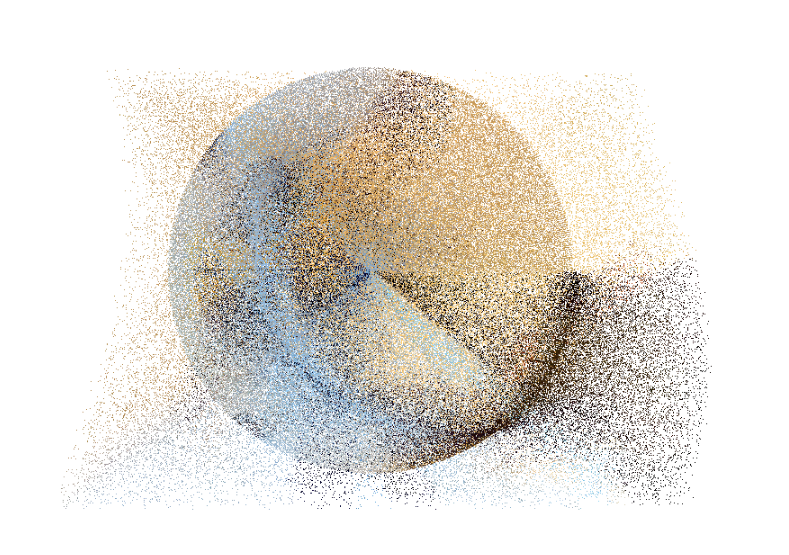# Tweening Particles With Three.js

I had this idea to use the concept of spherical projection to make a nifty thing with the webcam and particles.The end result is meh-okayish. Play with it here

–Process–

My first go at it. Rather underwhelming, would it be more interesting with particle tweening as seen in this Three.js example?

Second go at it. Switched to a buffer geometry and it’s much faster.

Third go at it. Wanted to tween the particles, and would’ve been impossible to tween all 30,000 using the CPU. So switched to a shader material in order to use the GPU for the calculations. Limitations of this implementation, I was calling Tween once and sharing its value (via a shader material uniform) with all the particles, which resulted in all the particles moving identically.

Fourth time is the charm. I moved all the tween logic to the shader (both the easing and interpolation functions). And yay, per pixel tweening! Also, it’s quiet and fast! (My laptop’s fans are usually spinning wildly when running a Three.js app).

Some psuedocode. See `view-source` for full code.

``````
// Buffer Geometry

var geometry = new THREE.BufferGeometry();
geometry.addAttribute( 'position', new THREE.BufferAttribute( bPositions, 3 ) );
geometry.addAttribute( 'color', new THREE.BufferAttribute( bColors, 3 ) );
geometry.addAttribute( 'targetPosition', new THREE.BufferAttribute( bPositions2, 3 ) );

uniforms: {
elapsedTime : {
type: "f",
value: 0.0
},
duration : {
type: "f",
value: 0.0
}
},
});

uniform float elapsedTime;
uniform float duration;
attribute vec3 targetPosition;

float exponentialInOut( float k ){
// https://github.com/tweenjs/tween.js/blob/master/src/Tween.js
if( k <= 0.0 ){
return 0.0;
}
else if( k >= 1.0 ){
return 1.0;
}
else if( ( k *= 2.0 ) < 1.0 ){
return 0.5 * pow( 1024.0, k - 1.0 );
}
return 0.5 * ( - pow( 2.0, - 10.0 * ( k - 1.0 ) ) + 2.0 );
}

void main(){

// calculate time value (also vary duration of each particle)
float t = elapsedTime / ( duration * ( 1.0 + randomNum.x ) );

// calculate progress
float progress = exponentialInOut( t );

// calculate new position (simple linear interpolation)
vec3 delta = targetPosition - position;
vec3 newPosition = position + delta * progress;

// something
gl_Position = projectionMatrix * modelViewMatrix * vec4( newPosition, 1.0 );
}
``````

Takeaways. I now know how to make projects run quietly and fast on Three.js. I also have a better understanding of shader advantages and limitations.